# 【專利讓與】用於治療中風的環烯醚萜苷類化合物，其醫藥組合物及其用途

 中國醫藥大學 研發成果讓與公告 本校案件 科技部成果公告讓與 公告日期 2021/06/01 發明人代表 中國醫藥大學 生物醫學研究所 徐偉成教授 專利(技術)名稱 用於治療中風的環烯醚萜苷類化合物，其醫藥組合物及其用途 專利國別 美國 專利核准日 2018.07.31 專利證號 US 10,035,791 B2 類型 發明 專利範圍 1. A bicyclic compound represented by: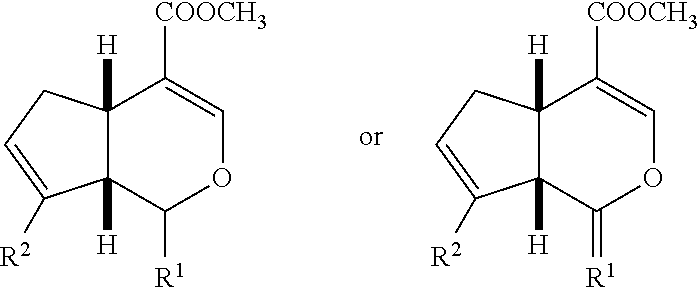wherein —R1 is —OH, —OCH2CH3, a substituent represented by Formula (i), a substituent represented by Formula (ii), a substituent represented by Formula (iii) or a substituent represented by Formula (iv);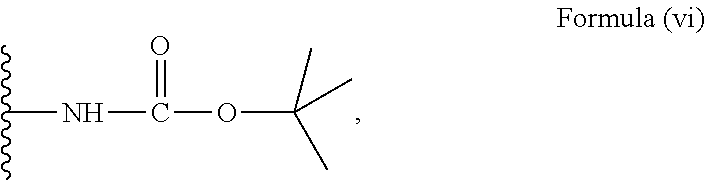wherein R2 is a substituent represented by Formula (vii):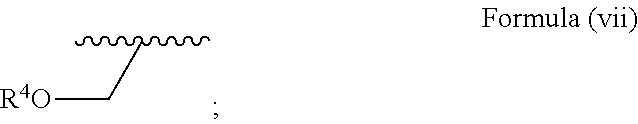wherein R4 is a substituent represented by Formula (viii), a substituent represented by Formula (ix) or a substituent represented by Formula (x):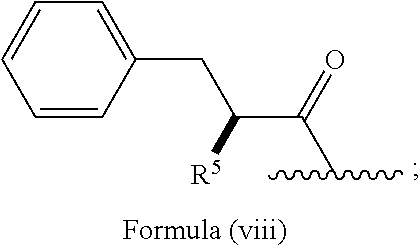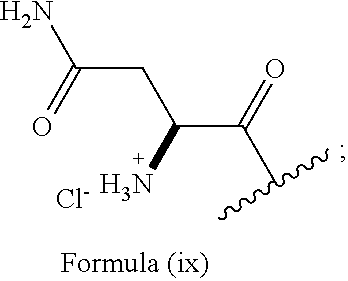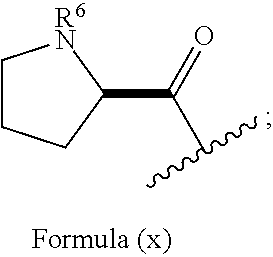wherein in Formula (viii), R5 is —NH2, —NH3 +Cl−or the substituent represented by Formula (vi);and in Formula (x), R6 is —H2 +Cl−or the substituent represented by Formula (vi).3. The bicyclic compound of claim 1 represented by a structural formula 1: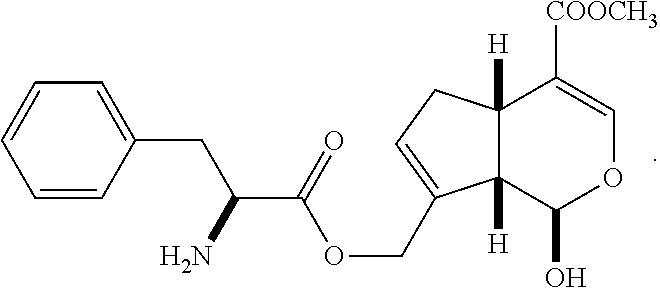4. The bicyclic compound of claim 1 represented by a structural formula 2: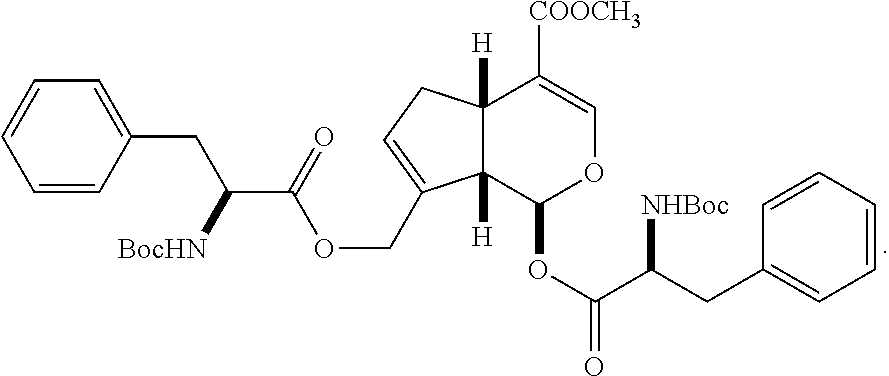5. The bicyclic compound of claim 1 represented by a structural formula 3: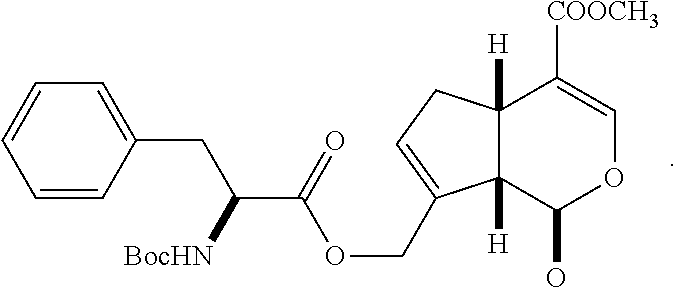6. The bicyclic compound of claim 1 represented by a structural formula 4: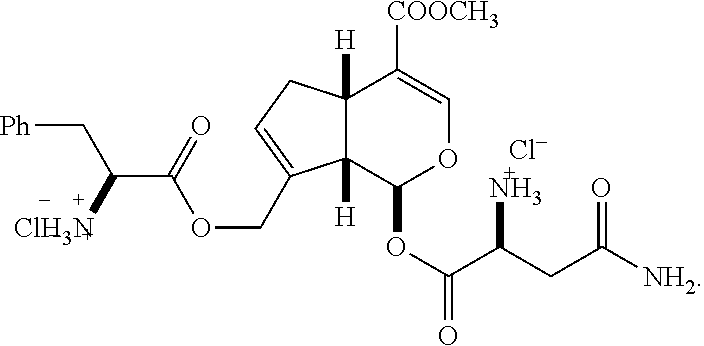7. The bicyclic compound of claim 1 represented by a structural formula 5: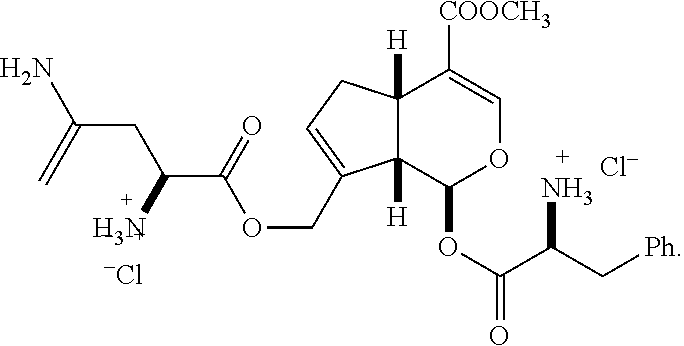8. The bicyclic compound of claim 1 represented by a structural formula 6: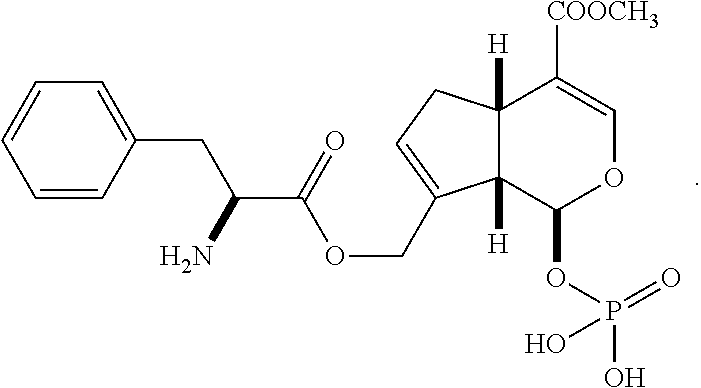9. The bicyclic compound of claim 1 represented by a structural formula 7: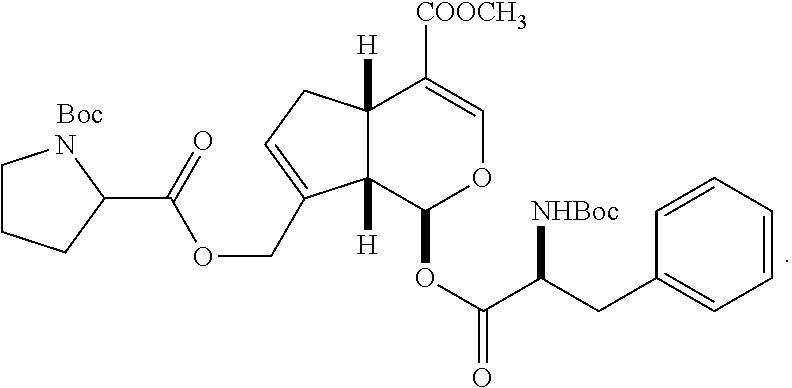10. The bicyclic compound of claim 1 represented by a structural formula 8: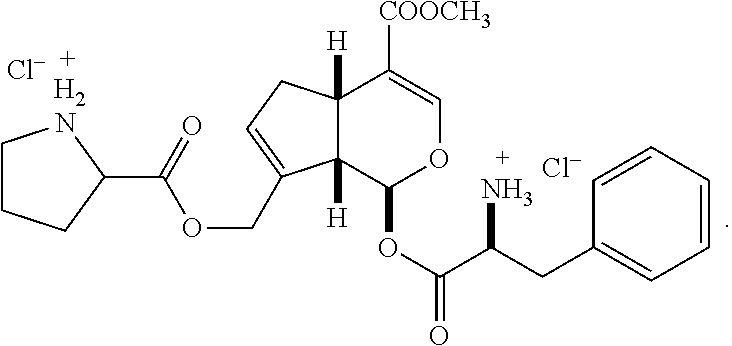11. The bicyclic compound of claim 1 represented by a structural formula 9: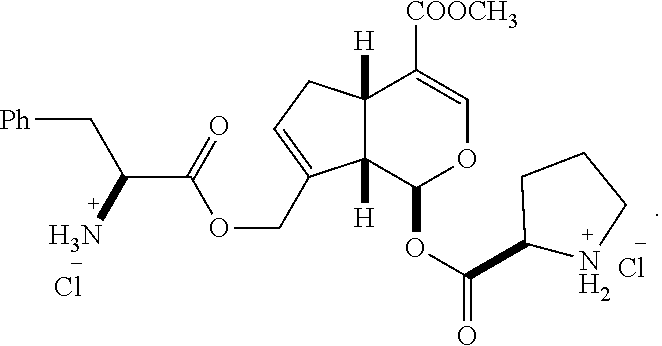12. The bicyclic compound of claim 1 represented by a structural formula 10: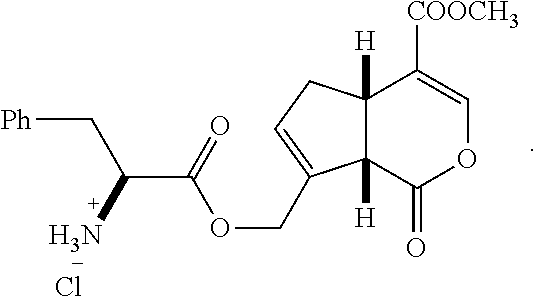13. The bicyclic compound of claim 1 represented by a structural formula 11: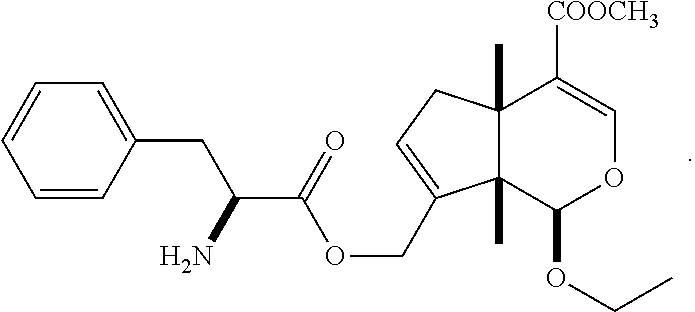14. The bicyclic compound of claim 1 represented by a structural formula 12: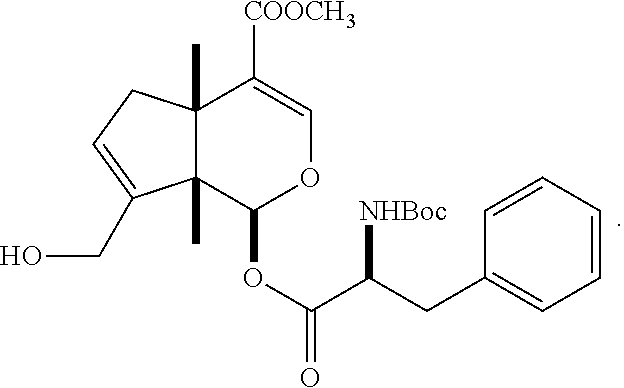15. The bicyclic compound of claim 1 represented by a structural formula 14: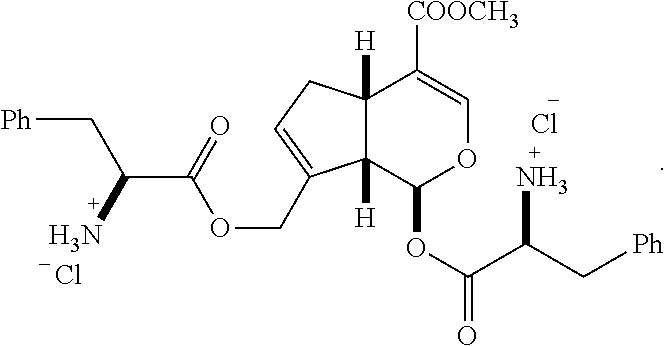16. The bicyclic compound of claim 1 represented by a structural formula 15: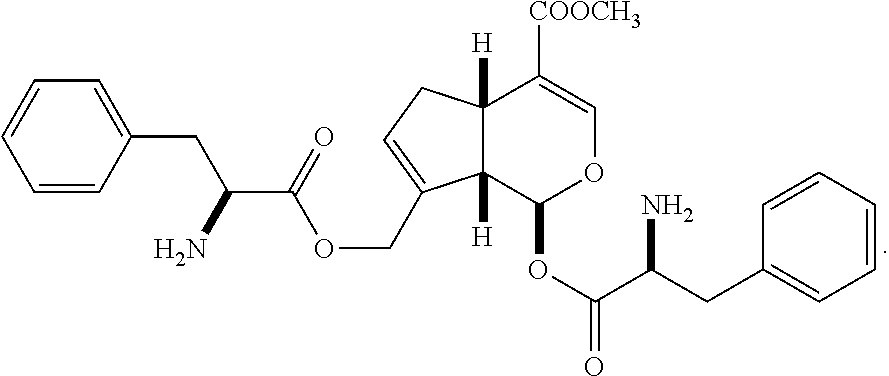17. The bicyclic compound of claim 1 represented by a structural formula 16: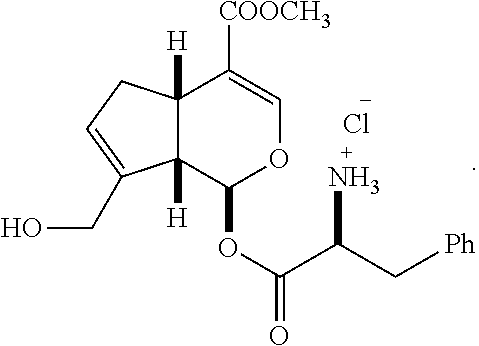18. The bicyclic compound of claim 1 represented by a structural formula 17: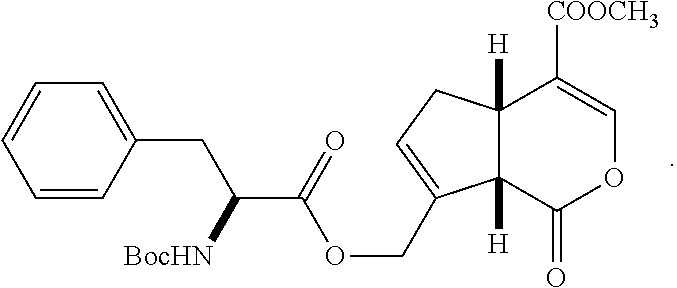19. A pharmaceutical composition for treating a stroke comprising an effective amount of the bicyclic compound of claim 1 and a pharmaceutically acceptable carrier, wherein the effective amount is from 0.1 mg/kg to 10 mg/kg.20. A method for treating an ischemic stroke comprising administering an effective amount of the bicyclic compound of claim 1 to a subject in need for a treatment of the stroke.